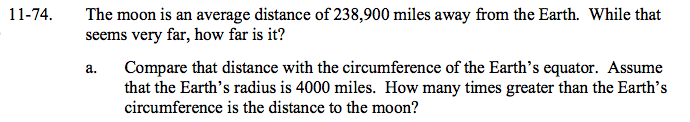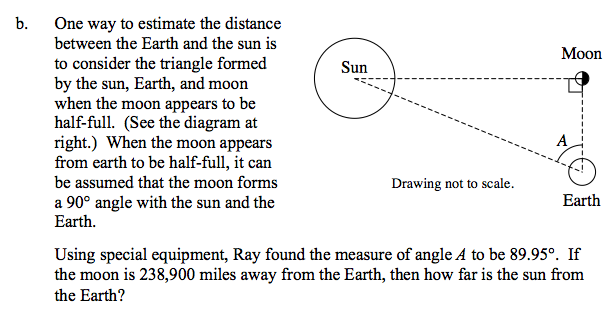### Home > GC > Chapter 11 > Lesson 11.2.1 > Problem11-74

11-74.The formula to find the circumference of a circle is 2πr.

≈ 9.5 times greater$\text{cos }\angle A =\frac{\text{distance between Earth and Moon}}{\text{distance between Earth and Sun}}$

≈ 91,253,182.4 miles